# Which row of the table is correct? Increasing number of carbon atoms —A)Ethyl methanote Methyl propanoatePentyl pentanoate Propyl butanoateB)ethyl methanoatemethyl propanoatepropyl butanoatepentyl pentanoateC)methyl propanoatepropyl butanoateethyl methanoatepentyl pentanoateD)propyl butanoateethyl methanoatepentyl pentanoatemethyl propanoate A) A B) B C) C D) DVerified
182.1k+ views
Hint: Esters are organic compounds. These compounds have a functional group $\text{ }-\text{COOR}$ . It is derived from the acid where $\text{ }-\text{OH }$that is the hydroxyl group is replaced by the alkoxy $\text{ }-\text{OR}$ group. The general representation of ester is as follows,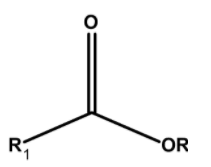The IUPAC nomenclature involves the two parts. The compound is visually separated from the oxygen atom adjacent to the carbonyl group. The group which is from the acid part is ended by the –oate.

The ester is the organic compound. To determine the name of a compound. Follows these steps:
1) First identify the carbon which is the part of the continuous chin which is bonded to the carbon on both sides (one from alkyl and the other from carboxyl).
2) Then start the numbering by considering the oxygen on either side.
3) The general format is,
(Alkyl on the side further from the carbonyl) (Alkane on the other side of carbonyl)
4) Replace the ending of alkane .change the –e to the -oate.
Here we are interested to determine the increasing number of carbon atoms in the compounds.
The structure of ethyl methanoate has ethyl groups $\text{ }-{{\text{C}}_{\text{2}}}{{\text{H}}_{\text{5}}}\text{ }$and $\text{ }-\text{COC}{{\text{H}}_{\text{3}}}\text{ }$ on either side of the oxygen. Thus the structure of ethyl methanoate is as follows,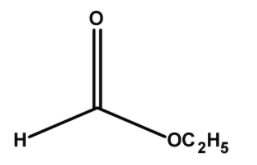Thus, the ester ethyl methanoate has a total $\text{ 2 + 1 = 3 }$ number of carbon atoms.
The structure of methyl propanoate has ethyl groups $\text{ }-\text{C}{{\text{H}}_{3}}\text{ }$and $\text{ }-\text{COC}{{\text{H}}_{2}}\text{C}{{\text{H}}_{\text{3}}}\text{ }$ on either side of the oxygen. Thus the structure of ethyl methanoate is as follows,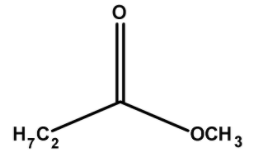Thus, the ester methyl propanoate has a total $\text{ 1 + 3 = 4 }$ number of carbon atoms.
The structure of propyl butanoate have propyl groups $\text{ }-\text{C}{{\text{H}}_{2}}\text{C}{{\text{H}}_{\text{2}}}\text{C}{{\text{H}}_{\text{3}}}\text{ }$and $\text{ }-\text{COC}{{\text{H}}_{2}}\text{C}{{\text{H}}_{2}}\text{C}{{\text{H}}_{\text{3}}}\text{ }$ on either side of the oxygen. Thus the structure of ethyl methanoate is as follows,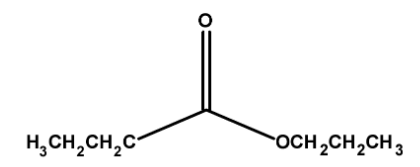Thus, the ester methyl propanoate has a total $\text{ 3 + 4 = 7 }$ number of carbon atoms.
The structure of pentyl pentanoate has pentyl groups $\text{ }-\text{C}{{\text{H}}_{2}}\text{C}{{\text{H}}_{\text{2}}}\text{C}{{\text{H}}_{2}}\text{C}{{\text{H}}_{\text{2}}}\text{C}{{\text{H}}_{\text{3}}}\text{ }$and $\text{ }-\text{COC}{{\text{H}}_{2}}\text{C}{{\text{H}}_{2}}\text{C}{{\text{H}}_{2}}\text{C}{{\text{H}}_{\text{3}}}\text{ }$ on either side of the oxygen. Thus the structure of ethyl methanoate is as follows,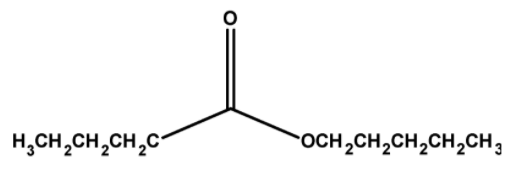Thus, the ester methyl propanoate has a total $\text{ 5 + 5 = 10 }$ number of carbon atoms.
Thus correct order of increasing number of carbon atom is,
$\text{ }\begin{matrix} \text{Ethyl methanoate }<\text{ Methyl propanoate }<\text{ propyl butanoate }<\text{ pentyl pentanoate} \\ (\text{Increasing number of carbon atom }\to \text{)} \\ \end{matrix}$

Hence, (B) is the correct option.

Note:
Note that esters are derived from the carboxylic acid. In naming the compound, visually we can see alcohol and the acid in the ester. The part which has the acid groups is named as the acid (-ic) and the –ic is replaced by the suffix –ate. The alkyl groups of the alcohol part are written in front of the acid part.to draw the structure, first, write down the $\text{ }-\text{O}-\text{C}=\text{O}$ groups and attached the alkyl part on the oxygen atom and –ate ending carbon atoms on the carboxyl carbon atoms.